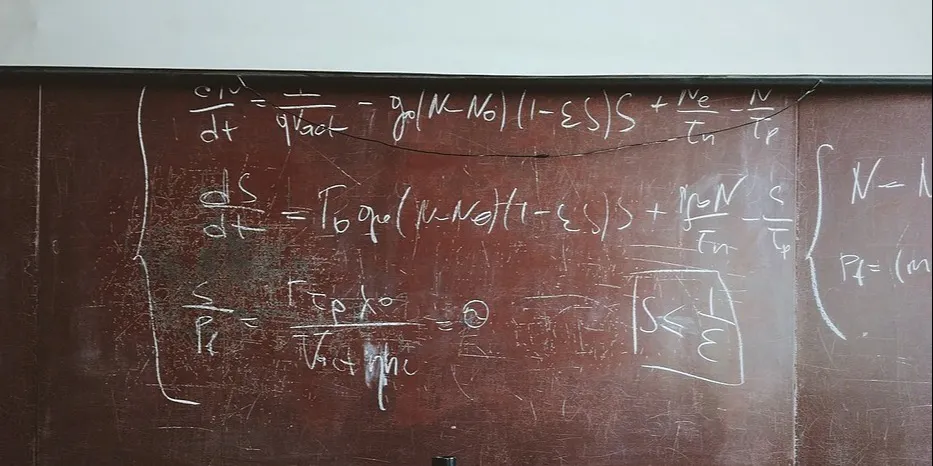Calculate Your Crypto Taxes using the Formulae Below OR Simply Use My Automated Softwareby@robinyo

# Calculate Your Crypto Taxes using the Formulae Below OR Simply Use My Automated Software

August 10th 2019
5 min🖨️

Calculate Your Crypto Taxes using the Formulae Below or Simply Use My Automated Software. The amount of tax you pay is a percentage of your total capital gains. A capital gain (profit/loss) occurs when you sell or trade cryptocurrencies and is calculated by subtracting the price you bought the crypto for (cost-basis) from the price that you are selling it for. This seemingly simple formula becomes complicated when you add more transactions as we will see. The best and easiest way of explaining the process of calculating your capital gains is through an example.### @robinyo

Robin Singh